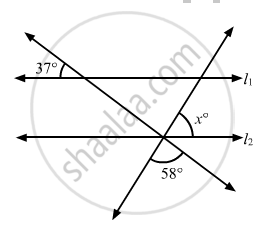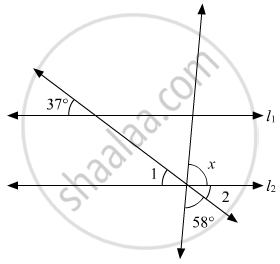# In the Given Figure, If L1 || L2, What is the Value of X? - Mathematics

MCQ

In the given figure, if l1 || l2, what is the value of x?• 80°

• 85°

• 90°

• 95°

#### Solution

In the given figure:Since, l1 || l2therefore, the pair of corresponding angles should be equal.

That is;

∠1 = 37°

Also,

$\angle1\text { and } \angle2$ are vertically opposite angles, therefore,

∠2 = ∠1

∠2 = 37°

Since 58°, ∠2 and x form a linear pair. Therefore,

58 +∠2 + x = 180°

58° + 37° | x = 180°

95° + x = 180°

x = 180° - 95°

x = 85°

Concept: Pairs of Angles
Is there an error in this question or solution?
Chapter 10: Lines and Angles - Exercise 10.6 [Page 53]

#### APPEARS IN

RD Sharma Mathematics for Class 9
Chapter 10 Lines and Angles
Exercise 10.6 | Q 14 | Page 53

Share